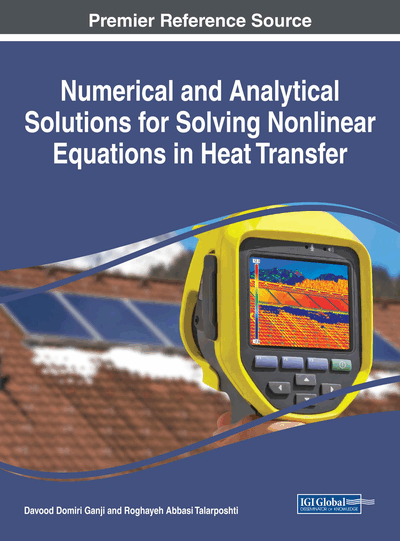# Akbari-Ganji's Method

DOI: 10.4018/978-1-5225-2713-8.ch009
Available
\$37.50
No Current Special Offers

## Abstract

The nonlinear partial differential equation governing on the mentioned system has been investigated by a simple and innovative method which we have named it Akbari-Ganji's Method or AGM. It is notable that this method has been compounded by Laplace transform theorem in order to covert the partial differential equation governing on the afore-mentioned system to an ODE and then the yielded equation has been solved conveniently by this new approach (AGM). One of the most important reasons of selecting the mentioned method for solving differential equations in a wide variety of fields not only in heat transfer science but also in different fields of study such as solid mechanics, fluid mechanics, chemical engineering, etc. in comparison with the other methods is as follows: Obviously, according to the order of differential equations, we need boundary conditions so in the case of the number of boundary conditions is less than the order of the differential equation, this method can create additional new boundary conditions in regard to the own differential equation and its derivatives. Therefore, a solution with high precision will be acquired. With regard to the afore-mentioned explanations, the process of solving nonlinear equation(s) will be very easy and convenient in comparison with the other methods.
Chapter Preview
Top

## The Analytical Method

Boundary conditions and initial conditions are required for analytical methods of each linear and nonlinear differential equation according to the physic of the problem. Therefore, we can solve every differential equation with any degrees. In order to comprehend the given method in this paper, two differential equations governing on engineering processes will be solved in this new manner.

In accordance with the boundary conditions, the general manner of a differential equation is as follows:

(1)

Boundary conditions:

(2)

To solve the first differential equation with respect to the boundary conditions inin Eq. (2), the series of letters in theth order with constant coefficients which is the answer of the first differential equation is considered as follows:

(3)

The more precise answer of Eq. (1), the more choice of series sentences from Eq. (3). In applied problems, approximately five or six sentences from the series are enough to solve nonlinear differential equations. In the answer of differential Eq. (3) regarding the series from degree, there areunknown coefficients that needequations to be specified. The boundary conditions of Eq. (2) are used to solve a set of equations which is consisted ofones.

## Complete Chapter List

Search this Book:
Reset